# Deep Neural Network Hyper-Parameter OptimizationRescale’s Design-of-Experiments (DOE) framework is an easy way to optimize the performance of machine learning models. This article will discuss a workflow for doing hyper-parameter optimization on deep neural networks. For an introduction to DOEs on Rescale, see this webinar.

Deep neural networks (DNNs) are a popular machine learning model used today in many many applications including robotics, self-driving cars, image search, facial recognition, and speech recognition. In this article, we will train some neural networks to do image classification and show how to use Rescale to maximize the performance of your DNN models.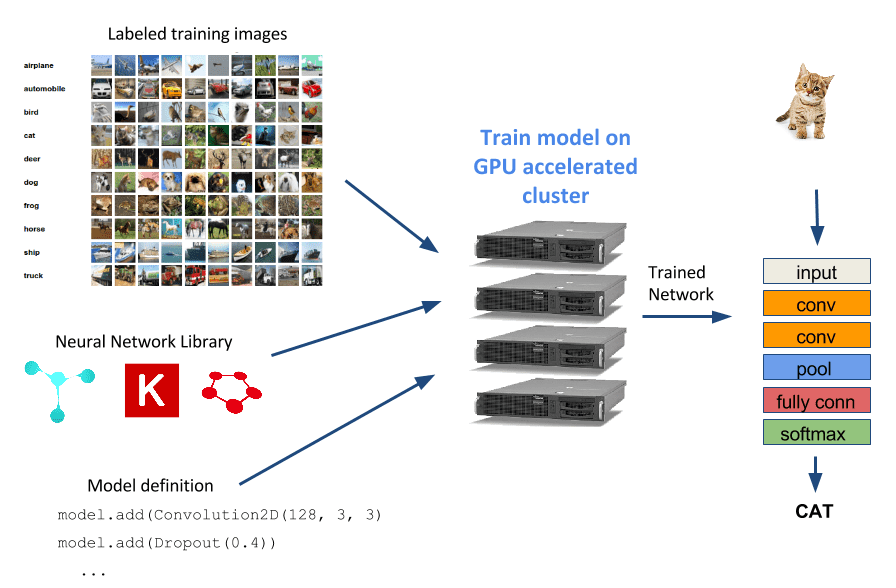For an introduction on training an image classification DNN on Rescale, please see this previous article.  This article will expand on the basic model training example and show how to improve the performance of your network through a technique called hyper-parameter optimization.
Hyper-Parameter Optimization
A typical starting point for a task using DNNs is to select a model published in the literature and implement it in the neural network training framework of your choice, or even easier, download an already-implemented model from the Caffe Model Zoo.  You might then train the model on your training dataset and find that the performance (classification accuracy, training time, etc.) is not quite good enough.  At this point, you could go back and look for a whole new model to train, or you can try tweaking your current model to get the additional performance you desire.  The process of tweaking parameters for a given neural network architecture is known as hyper-parameter optimization.  Here is a brief list of hyper-parameters people often vary:

• Learning rates
• Batch size
• Training epochs
• Image processing parameters
• Number of layers
• Convolutional filters
• Convolutional kernel size
• Dropout fractionsGiven the large list hyper-parameter choices above, even once we set the model architecture, there are still a large number of similar neural network variants.  Our task is to find a variant that performs well enough for our needs.
Randomized Hyper-Parameter Search DOE
Using the Rescale platform, we are now going to create a Design-of-Experiments job to sample hyper-parameters and then train these different network variants using GPUs.

For our first example, we will start with one of the example convolutional networks from the Keras github repository to train an MNIST digit classifier.  Using this network, we will vary the following network parameters:

• nb_filters: Number of filters in our convolutional layer
• nb_conv_size: Size of convolutional kernel

We modified the example to have the template values above and here is an excerpt from the script with the templated variables:

```model.add(Convolution2D(\${nb_filters}, \${nb_conv_size},
\${nb_conv_size}))

Note the nb_filters and nb_conv_size parameters surrounded by \${}.  We are now ready to create a DOE job on the Rescale platform that uses this template.
If you want to follow along, you can clone the example job:
Keras MNIST DOEFirst, we select the DOE job type in the top right corner and upload the mnist.pkl.gz dataset from the Keras github repository.Next, we specify that we are running a Monte Carlo DOE (lower left), that we want to do 60 different training runs, and we specify our template variables. We somewhat arbitrarily choose both parameters to be sampled from a uniform distribution. The convolutional kernel size ranges from 1 to 10 (each image size is 28×28, so more than 28 would not work), and the number of convolutional filters ranges from 16 to 256.

Note, we could specify our template variable ranges with a CSV instead. For this example, we would manually sample random values for our variables and the CSV would look like this:

```Nb_filters, nb_conv_size
16, 3
45, 8
32, 4
...```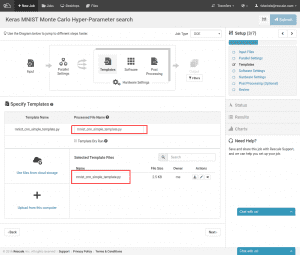Next, we need to upload the Keras script template that we created above.  At this point, we can also specify a new name for the script with the values inserted.  It is also fine if you just want to keep the name the same, as we have done here.  The materialized version will not overwrite the template.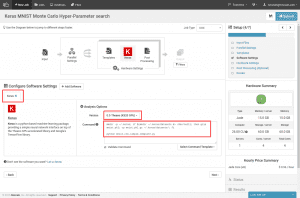Time to select the training library we want to use. Search for “Keras” in the search box and select it. Then, we select the K520/Theano compatible version.  Finally, we enter the command line to run the training script.  The first part of the command is just copying the MNIST dataset archive into a place where Keras can find it.  Then, we are just calling the python script.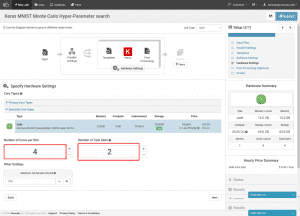Since we selected a version of Keras configured to work with K520s, our hardware type is already narrowed down to Jade.  We can now size each training cluster on the left.  The count here is in number of CPU cores.  For the Jade hardware type, there are 4 CPU cores for every GPU.  On the right, we set the number of separate training clusters we will provision.  In this case we are using 2 training clusters with a single GPU per cluster.The last step is to specify the post-processing script.  This script is used to parse the output of our training jobs and make any metrics available to Rescale to show in the results page we will see later.  The expected output format is a line for each value:

`[name]\t[value]`

Since the training script already prints the properly formatted accuracy as the last line of its output, result.out, our post-processing script just needs to parse out that last line.
You can now submit the job in the upper right and we move to the real-time cluster status page.Once the clusters are provisioned and the job starts, we can view the progress of our training jobs.  In the live tailing window, select a run and select process_output.log.  If the training has already started, you can view the training progress and the current training accuracy.  Individual runs can be terminated manually by selecting the “x” next to the select run number.  This enables the user to stop a run early if the parameters are clearly yielding inferior accuracy.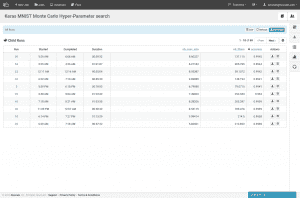Once our job completes, the results page summarizes the hyper-parameters used for each run and the accuracy results.  In the above case, the initial model we took from the Keras examples had an accuracy of 99.1% and the best result we obtain has an accuracy of about 99.4%, a small improvement.  If we want to download the weights for the most accurate model, we can sort by accuracy and then select the run details.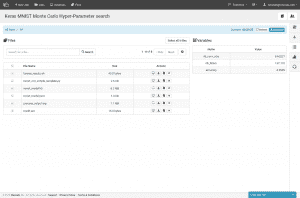Among other results files is mnist_model.json and mnist_model.h5, which are the model architecture and model weights files needed to reload the model back into Keras.  We can also download all the data from all runs as one big archive or download the results table as a CSV.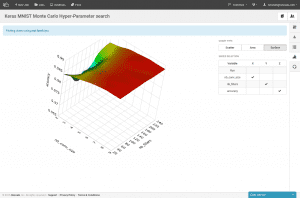Our accuracy results can also be visualized in the charting tab.
Rescale supports the use of 3rd party optimization software as detailed here. We will now discuss creating a Rescale optimization job to run a black-box optimizer from the machine learning literature.

Using the Rescale optimization SDK, we choose to plug in the
Sequential Model-based Algorithm Configuration (SMAC) optimizer from the University of British Columbia. The SMAC optimizer builds a random forest model of our neural network performance, based on the hyper-parameter choices. We use version 2.10.03, available here.

The optimizer is a Java application which takes the following configuration:

• “scenario” file: specifies the command line for the optimizer to run, in this case this is our network training script
• parameter file: specifies the names of our hyper-parameters and ranges of values

When SMAC runs the training script, it passes the current hyper-parameter selections to evaluate as command line flags.  It expects to receive results from the run in stdout, formatted as a string like this:

Result of this algorithm run: , , , , ,
To start, we create a parameter file for the hyper-parameters we will vary in this experiment:

```nb_filters integer [4, 256] 
nb_conv integer [1, 20] 
nb_pool integer [1, 20] 
dropout1 real [0, 1] [0.25]
dropout2 real [0, 1] [0.5]```

Now, in addition to varying the convolutional filter count and convolutional kernel size, we are also varying the dropout fractions and the size of the pooling layer.
Now we look at modifications made to our previous training script to accommodate SMAC input and results:

```parser = argparse.ArgumentParser()

Rather than injecting hyper-parameter values in as a template, we now just use argparse to parse the flags that are provided by SMAC.

```(X_train_orig, y_train_orig), (X_test, y_test) = mnist.load_data()
X_train = X_train_orig[:50000]
y_train = y_train_orig[:50000]
X_val = X_train_orig[50000:]
y_val = y_train_orig[50000:]```

Since we are feeding errors into an optimizer that then selects new parameters based on that error, we now keep separate validation and test datasets.  Above we are splitting the original training data into a training and validation set.  It is important to hold out a separate test dataset so we have an error metric to evaluate that is not in danger of being overfit by the optimizer.  The optimization algorithm only gets to see the validation error.

```  model.fit(X_train, Y_train, batch_size=batch_size, nb_epoch=nb_epoch,
show_accuracy=True, verbose=1, validation_data=(X_val, Y_val))
val_score = model.evaluate(X_val, Y_val, show_accuracy=True, verbose=0)
test_score = model.evaluate(X_test, Y_test, show_accuracy=True, verbose=0)
except Exception as e:
print(e)
print('Error running training')
satisfiable = False
finally:
json = model.to_json()
open('mnist_model.json', 'w').write(json)
model.save_weights('mnist_model.h5')
print('Test error:', 1 - test_score)
print('Val error:', 1 - val_score)
print('Result of algorithm run: {0}, {1}, {2}, {3}, {4},'.format(
'SAT' if satisfiable else 'UNSAT', time.time() - t0, 1, 1 - val_score, 1337
))```

Here we train the model, evaluate it on the validation and test datasets, and then output the results in the SMAC-specific format (“Result of algorithm…”).  Note, we also catch any errors in training and validation and mark that run as “UNSAT” to SMAC so that SMAC knows that it is an invalid combination of parameters.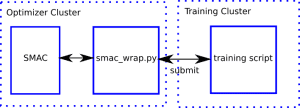In order for SMAC to call the Rescale python SDK, we write a wrapper script, which we will call smac_opt.py, and specify for SMAC to call it in the scenario file. The wrapper then submits the training script to be run.
Some important excerpts from the wrapper script:

```if __name__ == '__main__':
print objective_function(sys.argv[1:])```

To start, we take all the command line flags passed into the script and pass them directly to our objective function.  This is the objective function that will be called for each set of hyper-parameters.

```def objective_function(X):
iteration = os.getpid()
# Give each iteration its own files
run_dir = 'run-{0}'.format(iteration)
output_file = 'output-{0}'.format(iteration)
copy_input('input', run_dir)
# Let's package
zip_file = 'run.zip'
filezip(run_dir, zip_file)
# No need to keep directory on optimizer node
shutil.rmtree(run_dir)```

To start the objective function, we package up the input files for this training run into a .zip file.

```    COMMAND = 'python mnist_cnn_smac.py {0} | python format_results.py > {1}'
command = concatenate_shell_commands(
'unzip ' + zip_file,
'rm ' + zip_file,
'cd ' + run_dir,
COMMAND.format(' '.join(X), '../' + output_file),
'cd ..'
)
run = rescale.submit(
command,
input_files=[zip_file],
output_files=[output_file],
var_values=get_val_dict(X)
)
run.wait()```

Here, we format the training script command we will run.  Note, that we are just passing the flags from SMAC (variable `X`) through to the training script.  Then, we call the submit command which sends the input files to the training cluster and starts training.  We also now call the `format_results` script ourselves.

```# Get the objective function value from the output and save to Rescale
for line in open(output_file):
if re.match('Result of .*algorithm run.*', line):
results = line
m = re.match('Test error: ([\de\.-]+).*', line)
if m:
testerr = float(m.group(1))
quality = results.split(', ')
run.report({'testerr': float(testerr), 'valerr': float(quality)})
return results```

We parse the output file from the training script to get the expected SMAC results line (“Result of algorithm run…”), as well as the error on the test dataset.
Finally, we need to specify the scenario file that tells SMAC to call our wrapper script.

```use-instances = false
runObj = QUALITY
numberOfRunsLimit = 100
pcs-file = params.pcs
algo = ./smac_opt.py
check-sat-consistency = false
check-sat-consistency-exception = false```

The important parts here are:

• pcs-file: specifies the parameters
• algo: wrapper script to run
• numberOfRunsLimit: sets the number of training runs
• check-sat-consistency: tells the optimizer that for the same training dataset, different parameter selections might lead to a feasible or infeasible model
• So now that we have all of our input files, we are ready to create a job.

You can clone the job to run yourself here:
Keras MNIST SMAC Optimizer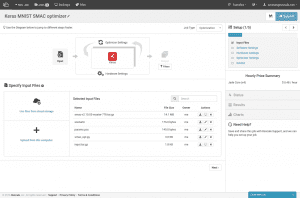The input.tar.gz archive consists of an input/ directory with our mnist_cnn_smac.py training script and the format_results.py post-processing script.
We select the same software as before, Keras configured for Theano on a K520.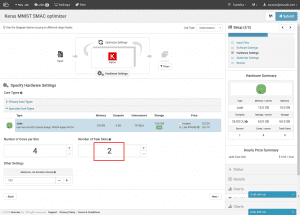Hardware selection is roughly the same as well. We again select 2 task slots to train 2 networks in parallel.For the optimizer, we select “Custom Optimization” and then enter the command line to run SMAC.  This command is made complicated by the fact that each SMAC process only runs one iteration at a time.  To run multiple trainings in parallel, we must use SMAC’s “shared model mode”.  Turning on this mode tells SMAC to periodically check its current directory for optimizer results from other SMAC processes and incorporate those results.  This mode requires we set the “-seed” to a different value for each SMAC process.
Since we need to run multiple optimizer processes at once, we background all the calls and then sleep for the maximum amount of time we would want to run this optimizer for.  In this case, we are waiting for 4 hours.
This job is now ready to submit.  Once running, you can live tail current iterations, and at the end, view results just as in the previous randomized case.  The results will now show both the validation and test errors for each set of hyper-parameters.
Conclusion
In this article, we showed 2 ways to search the space of hyper-parameters for a neural network.  One used randomized search and the other used a more sophisticated optimization tool, making use of the Rescale optimization SDK.

## Author

•Mark Whitney is a director of engineering at Rescale. His areas of expertise include high performance computing architectures, quantum information research, and cloud computing. He holds a PhD in computer science from the University of California, Berkeley